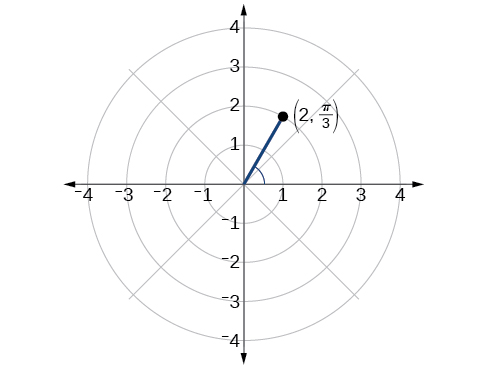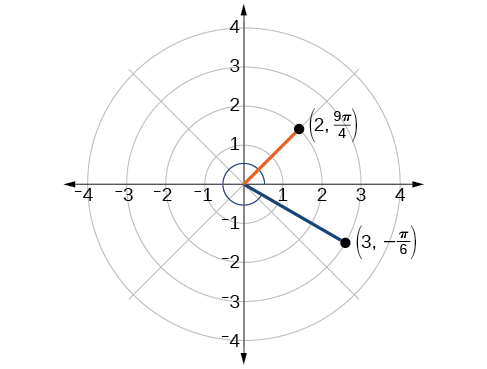# 8.3 Polar coordinates

 Page 1 / 8
In this section, you will:
• Plot points using polar coordinates.
• Convert from polar coordinates to rectangular coordinates.
• Convert from rectangular coordinates to polar coordinates.
• Transform equations between polar and rectangular forms.
• Identify and graph polar equations by converting to rectangular equations.

Over 12 kilometers from port, a sailboat encounters rough weather and is blown off course by a 16-knot wind (see [link] ). How can the sailor indicate his location to the Coast Guard? In this section, we will investigate a method of representing location that is different from a standard coordinate grid.

## Plotting points using polar coordinates

When we think about plotting points in the plane, we usually think of rectangular coordinates $\text{\hspace{0.17em}}\left(x,y\right)\text{\hspace{0.17em}}$ in the Cartesian coordinate plane. However, there are other ways of writing a coordinate pair and other types of grid systems. In this section, we introduce to polar coordinates    , which are points labeled $\text{\hspace{0.17em}}\left(r,\theta \right)\text{\hspace{0.17em}}$ and plotted on a polar grid. The polar grid is represented as a series of concentric circles radiating out from the pole    , or the origin of the coordinate plane.

The polar grid is scaled as the unit circle with the positive x- axis now viewed as the polar axis    and the origin as the pole. The first coordinate $\text{\hspace{0.17em}}r\text{\hspace{0.17em}}$ is the radius or length of the directed line segment from the pole. The angle $\text{\hspace{0.17em}}\theta ,$ measured in radians, indicates the direction of $\text{\hspace{0.17em}}r.\text{\hspace{0.17em}}$ We move counterclockwise from the polar axis by an angle of $\text{\hspace{0.17em}}\theta ,$ and measure a directed line segment the length of $\text{\hspace{0.17em}}r\text{\hspace{0.17em}}$ in the direction of $\text{\hspace{0.17em}}\theta .\text{\hspace{0.17em}}$ Even though we measure $\text{\hspace{0.17em}}\theta \text{\hspace{0.17em}}$ first and then $\text{\hspace{0.17em}}r,$ the polar point is written with the r -coordinate first. For example, to plot the point $\text{\hspace{0.17em}}\left(2,\frac{\pi }{4}\right),$ we would move $\text{\hspace{0.17em}}\frac{\pi }{4}\text{\hspace{0.17em}}$ units in the counterclockwise direction and then a length of 2 from the pole. This point is plotted on the grid in [link] .

## Plotting a point on the polar grid

Plot the point $\text{\hspace{0.17em}}\left(3,\frac{\pi }{2}\right)\text{\hspace{0.17em}}$ on the polar grid.

The angle $\text{\hspace{0.17em}}\frac{\pi }{2}\text{\hspace{0.17em}}$ is found by sweeping in a counterclockwise direction 90° from the polar axis. The point is located at a length of 3 units from the pole in the $\text{\hspace{0.17em}}\frac{\pi }{2}\text{\hspace{0.17em}}$ direction, as shown in [link] .

Plot the point $\text{\hspace{0.17em}}\left(2,\text{\hspace{0.17em}}\frac{\pi }{3}\right)\text{\hspace{0.17em}}$ in the polar grid .## Plotting a point in the polar coordinate system with a negative component

Plot the point $\text{\hspace{0.17em}}\left(-2,\text{\hspace{0.17em}}\frac{\pi }{6}\right)\text{\hspace{0.17em}}$ on the polar grid.

We know that $\text{\hspace{0.17em}}\frac{\pi }{6}\text{\hspace{0.17em}}$ is located in the first quadrant. However, $\text{\hspace{0.17em}}r=-2.\text{\hspace{0.17em}}$ We can approach plotting a point with a negative $\text{\hspace{0.17em}}r\text{\hspace{0.17em}}$ in two ways:

1. Plot the point $\text{\hspace{0.17em}}\left(2,\frac{\pi }{6}\right)\text{\hspace{0.17em}}$ by moving $\text{\hspace{0.17em}}\frac{\pi }{6}\text{\hspace{0.17em}}$ in the counterclockwise direction and extending a directed line segment 2 units into the first quadrant. Then retrace the directed line segment back through the pole, and continue 2 units into the third quadrant;
2. Move $\text{\hspace{0.17em}}\frac{\pi }{6}\text{\hspace{0.17em}}$ in the counterclockwise direction, and draw the directed line segment from the pole 2 units in the negative direction, into the third quadrant.

See [link] (a). Compare this to the graph of the polar coordinate $\text{\hspace{0.17em}}\left(2,\frac{\pi }{6}\right)\text{\hspace{0.17em}}$ shown in [link] (b).

Plot the points $\text{\hspace{0.17em}}\left(3,-\frac{\pi }{6}\right)$ and $\text{\hspace{0.17em}}\left(2,\frac{9\pi }{4}\right)\text{\hspace{0.17em}}$ on the same polar grid.## Converting from polar coordinates to rectangular coordinates

When given a set of polar coordinates    , we may need to convert them to rectangular coordinates . To do so, we can recall the relationships that exist among the variables $\text{\hspace{0.17em}}x,\text{\hspace{0.17em}}y,\text{\hspace{0.17em}}r,\text{\hspace{0.17em}}$ and $\text{\hspace{0.17em}}\theta .$

#### Questions & Answers

how fast can i understand functions without much difficulty
Joe Reply
what is set?
Kelvin Reply
a colony of bacteria is growing exponentially doubling in size every 100 minutes. how much minutes will it take for the colony of bacteria to triple in size
Divya Reply
I got 300 minutes. is it right?
Patience
no. should be about 150 minutes.
Jason
It should be 158.5 minutes.
Mr
ok, thanks
Patience
100•3=300 300=50•2^x 6=2^x x=log_2(6) =2.5849625 so, 300=50•2^2.5849625 and, so, the # of bacteria will double every (100•2.5849625) = 258.49625 minutes
Thomas
what is the importance knowing the graph of circular functions?
Arabella Reply
can get some help basic precalculus
ismail Reply
What do you need help with?
Andrew
how to convert general to standard form with not perfect trinomial
Camalia Reply
can get some help inverse function
ismail
Rectangle coordinate
Asma Reply
how to find for x
Jhon Reply
it depends on the equation
Robert
yeah, it does. why do we attempt to gain all of them one side or the other?
Melissa
whats a domain
mike Reply
The domain of a function is the set of all input on which the function is defined. For example all real numbers are the Domain of any Polynomial function.
Spiro
Spiro; thanks for putting it out there like that, 😁
Melissa
foci (–7,–17) and (–7,17), the absolute value of the differenceof the distances of any point from the foci is 24.
Churlene Reply
difference between calculus and pre calculus?
Asma Reply
give me an example of a problem so that I can practice answering
Jenefa Reply
x³+y³+z³=42
Robert
dont forget the cube in each variable ;)
Robert
of she solves that, well ... then she has a lot of computational force under her command ....
Walter
what is a function?
CJ Reply
I want to learn about the law of exponent
Quera Reply
explain this
Hinderson Reply

### Read also:

#### Get Jobilize Job Search Mobile App in your pocket Now!

Source:  OpenStax, Precalculus. OpenStax CNX. Jan 19, 2016 Download for free at https://legacy.cnx.org/content/col11667/1.6
Google Play and the Google Play logo are trademarks of Google Inc.

Notification Switch

Would you like to follow the 'Precalculus' conversation and receive update notifications?ByBy Darlene PaliswatBy Jazzycazz JacksonBy Nicole BartelsBy OpenStaxBy Subramanian DivyaBy Mahee BooBy Ryan LoweBy Abby SharpBy P. Wynn NormanBy Saylor Foundation